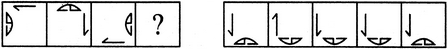# Non Verbal Reasoning - Analogy - Discussion

### Discussion :: Analogy - Section 1 (Q.No.18)

Each of the following questions consists of two sets of figures. Figures A, B, C and D constitute the Problem Set while figures 1, 2, 3, 4 and 5 constitute the Answer Set. There is a definite relationship between figures A and B. Establish a similar relationship between figures C and D by selecting a suitable figure from the Answer Set that would replace the question mark (?) in fig. (D).

18.

Select a suitable figure from the Answer Figures that would replace the question mark (?).

Problem Figures:                            Answer Figures:(A)     (B)      (C)     (D)                  (1)      (2)      (3)      (4)      (5)

 [A]. 1 [B]. 2 [C]. 3 [D]. 4 [E]. 5

Explanation:

Both the elements move to the adjacent side in a CW direction; the half-arrow rotates 90o ACW; the semi-circular element rotates 90oACW and gets laterally inverted.

 Subu said: (Jun 4, 2015) How's it possible? Can any one tell me?

 Jagadeesh said: (Aug 13, 2020) Not understanding, please explain.

 Adeeba Yousuf said: (Jun 12, 2022) @All. Look there is a relation between A and C so we should establish a relation between B and D. A and C are opposite to each other for the line but the half-circle will also be opposite.